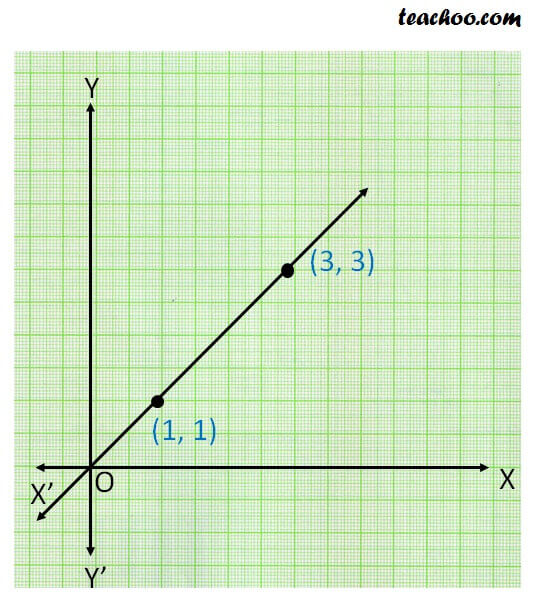Given two distinct points, there is a unique line that passes through

them.

In other words,

Only one lines passes through two distinct points1. Chapter 5 Class 9 Introduction to Euclid's Geometry (Deleted)
2. Concept wise
3. Axiom 5.1

Transcript

Axiom 5.1 Given two distinct points, there is a unique line that passes through them. Here, only one line passes through A & B